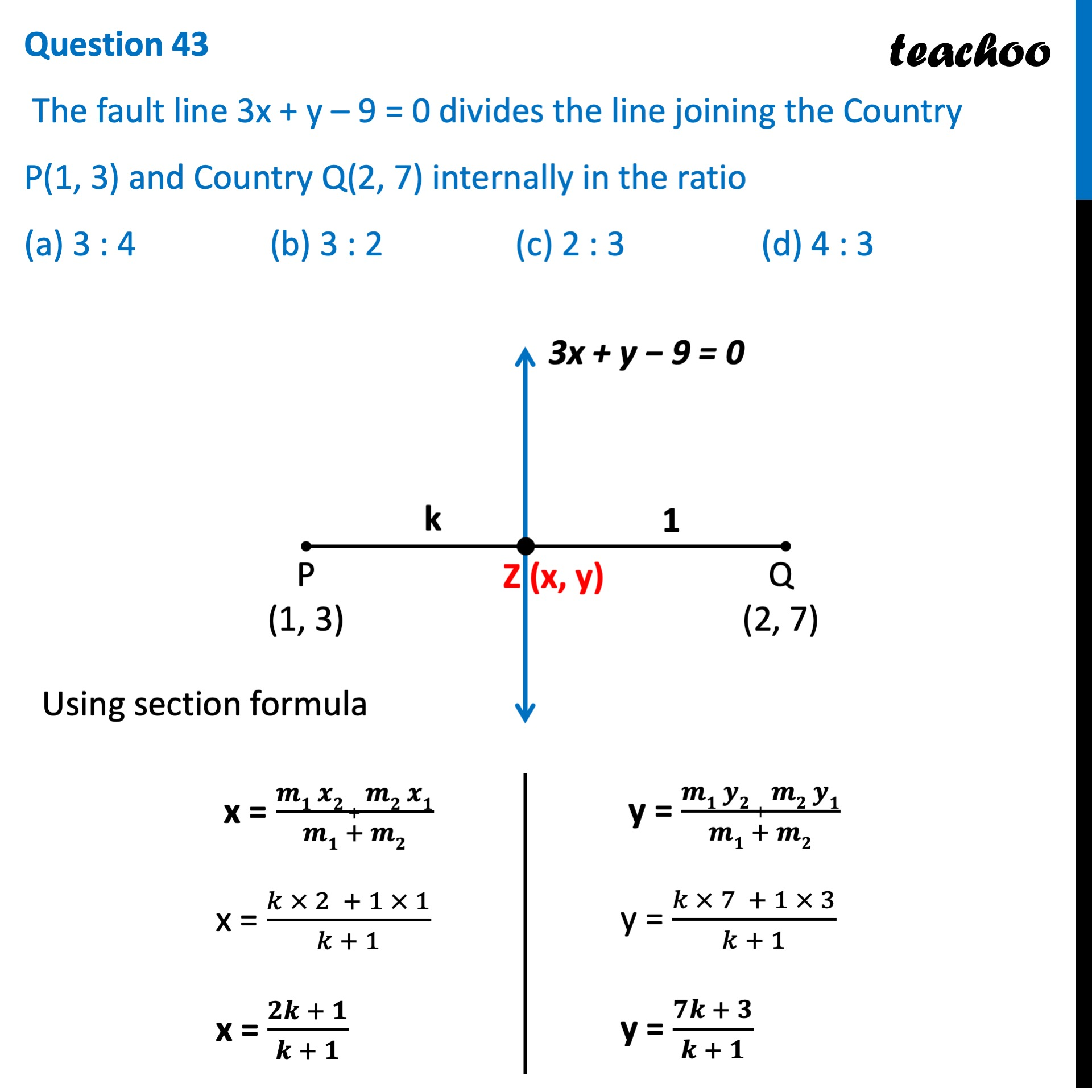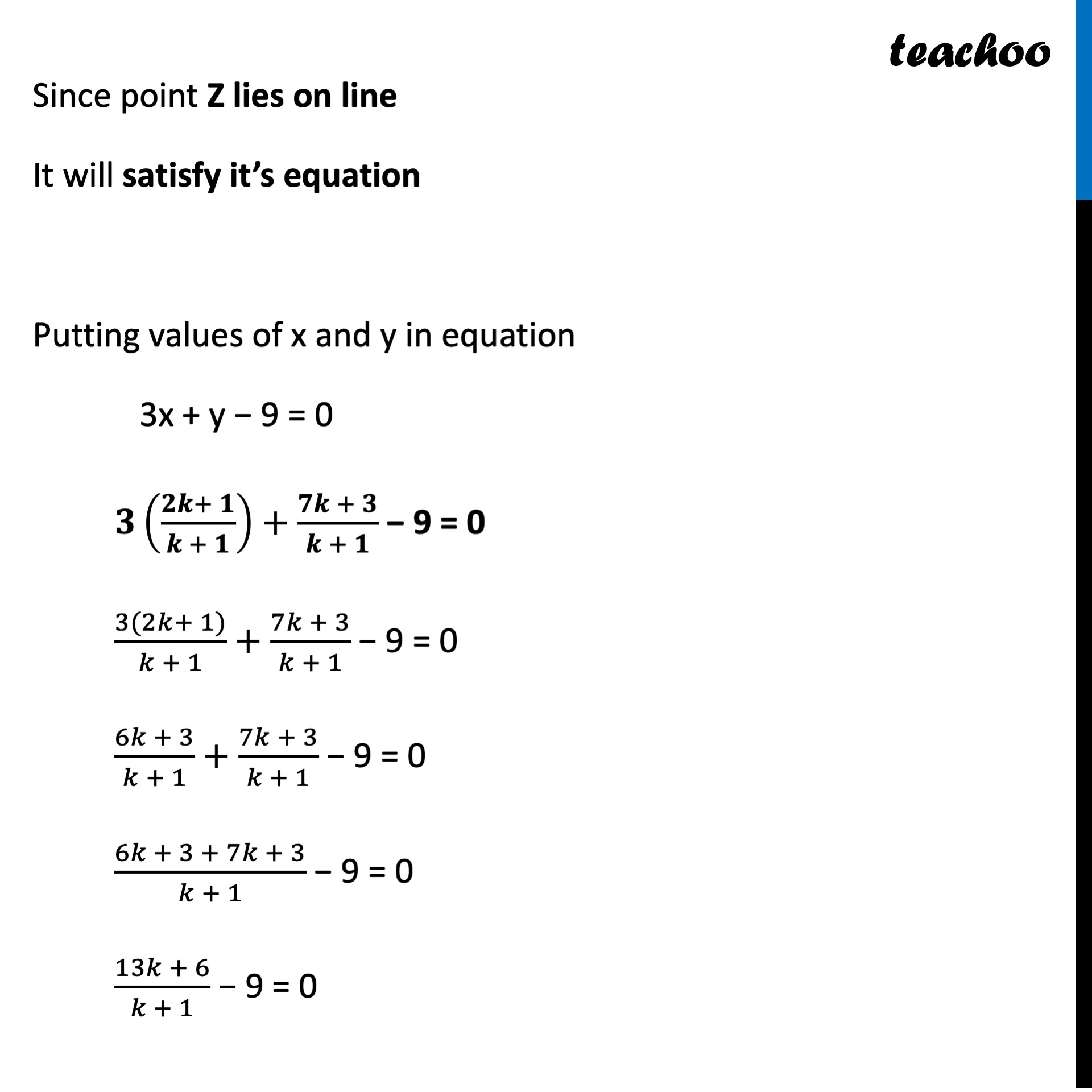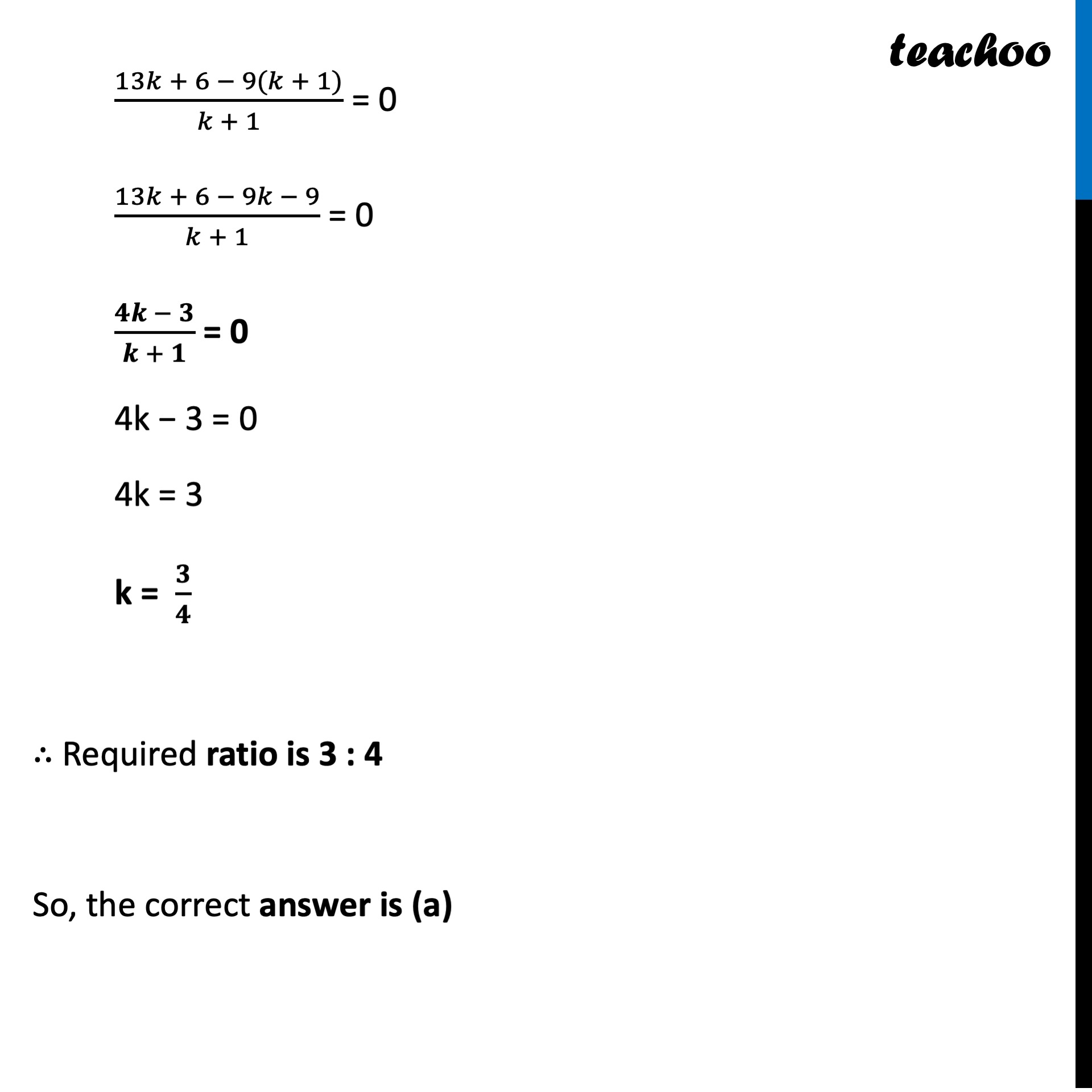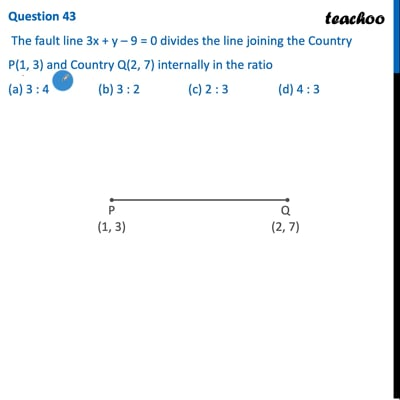CBSE Class 10 Sample Paper for 2022 Boards - Maths Basic [MCQ]

Class 10
Solutions of Sample Papers for Class 10 Boards

## (a) 3 : 4   (b) 3 : 2   (c) 2 : 3   (d) 4 : 3This video is only available for Teachoo black users

Learn in your speed, with individual attention - Teachoo Maths 1-on-1 Class

### Transcript

Question 43 The fault line 3x + y – 9 = 0 divides the line joining the Country P(1, 3) and Country Q(2, 7) internally in the ratio (a) 3 : 4 (b) 3 : 2 (c) 2 : 3 (d) 4 : 3 Using section formula x = (𝒎𝟏 𝒙𝟐 + 𝒎𝟐 𝒙𝟏)/(𝒎𝟏 + 𝒎𝟐) x = (𝑘 × 2 + 1 × 1)/(𝑘 + 1) x = (𝟐𝒌 + 𝟏)/(𝒌 + 𝟏) y = (𝒎𝟏 𝒚𝟐 + 𝒎𝟐 𝒚𝟏)/(𝒎𝟏 + 𝒎𝟐) y = (𝑘 × 7 + 1 × 3)/(𝑘 + 1) y = (𝟕𝒌 + 𝟑)/(𝒌 + 𝟏) Since point Z lies on line It will satisfy it’s equation Putting values of x and y in equation 3x + y − 9 = 0 𝟑((𝟐𝒌+ 𝟏)/(𝒌 + 𝟏))+(𝟕𝒌 + 𝟑)/(𝒌 + 𝟏) − 9 = 0 (3(2𝑘+ 1))/(𝑘 + 1)+(7𝑘 + 3)/(𝑘 + 1) − 9 = 0 (6𝑘 + 3)/(𝑘 + 1)+(7𝑘 + 3)/(𝑘 + 1) − 9 = 0 (6𝑘 + 3 + 7𝑘 + 3)/(𝑘 + 1) − 9 = 0 (13𝑘 + 6)/(𝑘 + 1) − 9 = 0 (13𝑘 + 6 − 9(𝑘 + 1))/(𝑘 + 1) = 0 (13𝑘 + 6 − 9𝑘 − 9)/(𝑘 + 1) = 0 (𝟒𝒌 − 𝟑)/(𝒌 + 𝟏) = 0 4k − 3 = 0 4k = 3 k = 𝟑/𝟒 ∴ Required ratio is 3 : 4 So, the correct answer is (a)